RS Aggarwal Class 10 Solutions Chapter 2 - Polynomials Ex 2A (2.1)

RS Aggarwal Class 10 Chapter 2 - Polynomials Ex 2A (2.1) Solutions Free PDF

The RS Aggarwal Class 10 Solutions prove to be one of the most important resources for exam preparation. These solutions of Chapter 10 Ex 2A (2.1) are provided in a detailed manner so that students can focus on important topics in a proper way. The solutions are prepared by our subject experts and refer these solutions to practice important questions and enhance your question-solving skills.

The topics mentioned in the Class 10 syllabus for Chapter 2 Polynomials are important and need to be prepared thoroughly. So, by utilizing our RS Aggarwal Solutions Class 10 Chapter 2 – Polynomials 2A (2.1) you can get detailed explanations for important topics and prepare the whole chapter thoroughly. So, practice these solutions and give yourself the opportunity to master the various concepts explained in Polynomial Chapter.

Download PDF of RS Aggarwal Solutions Class 10 Chapter 2– Polynomials Ex 2A (2.1)

Question 1: Find the cubic polynomial whose zeros are 3, ½, -1.

Solution:

Required polynomial = $(x-3)\left ( x-\frac{1}{2} \right )\left [ (x-(-1)) \right ]=(x-3)\left ( x-\frac{1}{2} \right )(x+1)$

= $(x-3)(x+1)\left ( x-\frac{1}{2} \right )=(x^{2}-2x-3)\left ( x-\frac{1}{2} \right )$

= $\frac{2x^{3}-4x^{2}-6x-x^{2}+2x+3}{2}$ = p(x)

p(x) = $\frac{2x^{3}-4x^{2}-6x-x^{2}+2x+3}{2}$

p(x) = $\frac{1}{2}(2x^{3}-5x^{2}-4x+3)$

Hence, required polynomial is $(2x^{3}-5x^{2}-4x+3)$

Question 2: On dividing 4x3 – 8x2 + 8x + 1 by a polynomial g(x), the quotient and the remainder are (2x – 1) and (x + 3) respectively. Find g(x).

Solution:

f(x) = 4x3 – 8x2 + 8x + 1

q(x) = (2x – 1)

r(x) = (x + 3)

By division algorithm, we have

Dividend = (Quotient x Divisor) + Remainder

f(x) = q(x) x g(x) + r(x)

(4x3 – 8x2 + 8x + 1) = (2x – 1) x g(x) + (x + 3)

g(x) = $\frac{(4x^{3}-8x^{2}+8x+1)-(x+3)}{(2x-1)}$

= $\frac{4x^{3}-8x^{2}+7x-2}{2x-1}=2x^{2}-3x+2$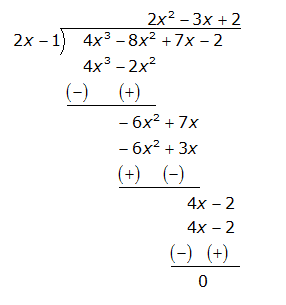Question 3: Divide 2x2 + x – 15 by x + 3 and verify the division algorithm.

Solution:

The terms of dividend and divisor are in decreasing order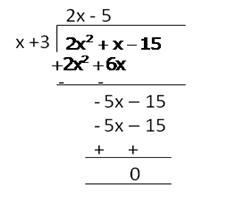Clearly degree (of remainder) = 0 < degree (x + 3)

Therefore, Quotient = 2x – 5 and remainder = 0

$\Rightarrow$ (Quotient x divisor) + remainder

= (2x – 5)(x + 3) + 0

= 2x2 + 6x – 5x – 15

= 2x2 + x – 15 = dividend

Thus, (Quotient x divisor) + remainder = dividend

Question 4: Divide -5x2 – 17x + 12 by -5x + 3 and verify the division algorithm.

Solution:

First, we write the terms of dividend and divisor in decreasing order of their degree and then perform the division as shown below.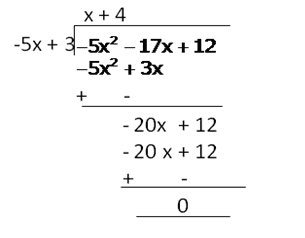Clearly degree (of remainder) = 0 < degree (-5x + 3)

Therefore, Quotient = x + 4 and remainder = 0

$\Rightarrow$ (Quotient x divisor) + remainder

= (x + 4)(-5x + 3) + 0

= -5x2 + 3x – 20x + 12 = 0

= -5x2 – 17x + 12 = dividend

Thus, (Quotient x divisor) + remainder = dividend

Hence, the division algorithm is verified.

Question 5: Divide 3x3 – 4x2 + 7x – 2 by x2 – x + 2 and verify the division algorithm.

Solution:

First, we write the given polynomials in standard form in decreasing order of degree and then perform the division as shown below.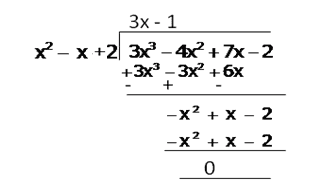Clearly degree (of remainder) = 0 < degree (x2 – x + 2)

Therefore, Quotient = (3x – 1), Remainder = 0

$\Rightarrow$ (Quotient x divisor) + remainder

= (3x – 1)(x2 – x + 2) + 0

= 3x3 – 3x2 + 6x – x2 + x – 2 = 0

= 3x3 – 4x2 + 7x -2 = dividend

Thus, (Quotient x divisor) + remainder = dividend

Hence, the division algorithm is verified.

Question 6:

Divide -6x3 + x2 + 19x + 6 by –3x2 + 5x + 2 and verify the division algorithm.

Solution:

First, we write the given polynomials in standard form in decreasing order of degree and then perform the division as shown below.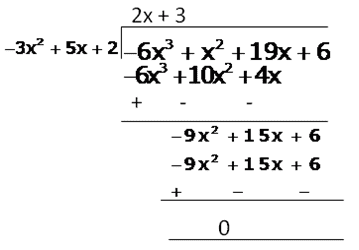Clearly degree (of remainder) = 0 < degree (-3x2 + 5x + 2)

Therefore, Quotient = (2x + 3), Remainder = 0

$\Rightarrow$ (Quotient x divisor) + remainder

= (2x + 3)(-3x2 + 5x + 2) + 0

= -6x3 + 10x2 + 4x – 9x2 + 15x + 6

Therefore, -6x3 + x2 + 19x + 6 = dividend

Thus, (Quotient x divisor) + remainder = dividend

Hence, the division algorithm is verified.

Question 7: It being given that 2 is a zero of the polynomial x3 – 4x2 + x + 6, find its other zeros.

Solution:

2 is the zero of the polynomial x3 – 4x2 + x + 6

Therefore, x – 2 is a factor of it

Dividing the given polynomial by x – 2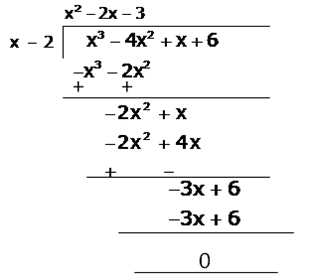Remainder = 0, Quotient of q(x) = x2 – 2x – 3

Now, q(x) = x2 – 2x – 3

= x2 – 3x + x – 3 = x(x – 3) + (x – 3)

= (x – 3)(x + 1)

Other zeros of given cubic polynomial are zeros of q(x)

Therefore, q(x) = 0 $\Rightarrow$ (x – 3)(x + 1) = 0

$\Rightarrow$ Either x – 3 = 0 or x + 1 = 0

Therefore, Either x  = 3 or x = -1

Therefore, Other zeros of the given cubic polynomial are 3 and -1

Question 8: It being given that -1 is a zero of the polynomial x3 + 2x2 – 11x – 12, find its other zeros.

Solution:

One zero of the polynomial x3 + 2x2 – 11x – 12 is -1

Therefore, x + 1 is a factor of x3 + 2x2 – 11x – 12

Dividing x3 + 2x2 – 11x – 12 by x + 1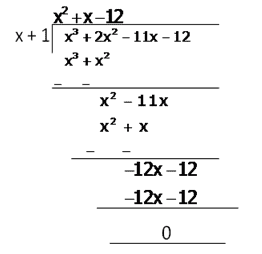Quotient q(x) = x2 + x – 12

= x2 + 4x – 3x – 12

= (x + 4)(x – 3)

Other zeros of given polynomial are the zeros of q(x)

Therefore, q(x) = 0

$\Rightarrow$ (x + 4)(x – 3) = 0

$\Rightarrow$ Either x + 4 = 0 or x – 3 = 0

$\Rightarrow$ Either x = -4 or x = 3

Therefore, -4, 3 are the zeros of q(x)

Therefore, The zeros of given polynomial are -4, -1 and 3

Question 9: If 1 and -2 are two zeros of the polynomial (x3 – 4x2 – 7x + 10), find its third zero.

Solution:

1 and -2 are the two zeros of the polynomial

x3 – 4x2 – 7x + 10

Dividing the polynomial by x – 1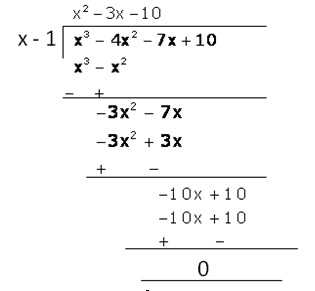Quotient q(x) = x2 – 3x – 10

2 is a zero of given polynomial so it is a zero of x2 – 3x – 10

Dividing x2 – 3x – 10 by x + 2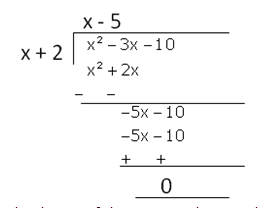Third zero of the given polynomial is given by x – 5 = 0 or x = 5

Question 10: If 3 and -3 are two zeros of the polynomial (x4 + x3 – 11x2 – 9x + 18), find all the zeros of the given polynomial.

Solution:

3 and -3 are the two zeros of the polynomial x4 + x3 – 11x2 – 9x + 18

Therefore, (x – 3)(x + 3) = x2 – 9 is a factor of given polynomial

Therefore, Dividing the given polynomial by x2 – 9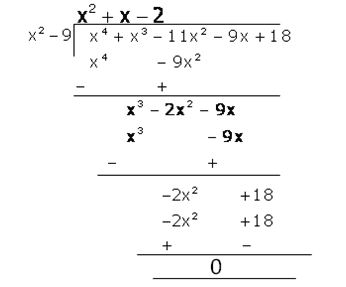Therefore, Quotient q(x) = x2 + x – 2

Other zeros of given polynomial are -2 and 1

So zeros of given polynomial are 1, -2,3 and -3

Question 11: If 2 and -2 are two zeros of the polynomial (x4 + x3 – 34x2 – 4x + 120), find all the zeros of the given polynomial.

Solution:

2 and -2 are two polynomials

x4 + x3 – 34x2 – 4x + 120

Therefore, (x – 2)(x + 2) = x2 – 4 will divide the given polynomial completely.

Dividing x4 + x3 – 34x2 – 4x + 120 by x2 – 4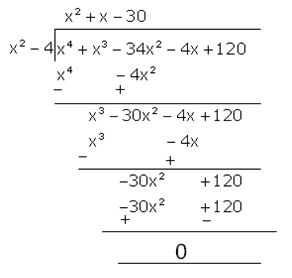Therefore, Quotient q(x) = x2 + x – 30

= x2 + 6x – 5x – 30

= x(x + 6) -5(x + 6)

= (x + 6)(x – 5)

For finding zeros of q(x), q(x) = 0

(x + 6)(x – 5) = 0, x = -6 or 5

Other zeros of given polynomial are -6 and 5

So zeros of given polynomial are 2, -2, -6 and 5

Question 12: Find all the zeros of (x4 + x3 – 23x2 – 3x + 60), if it is given that two of its zeros are $\sqrt{3}$ and $-\sqrt{3}$.

Solution:

$\sqrt{3}$ and $-\sqrt{3}$ are the zeros of polynomial

x4 + x3 – 23x2 – 3x + 60

Therefore, (x – $\sqrt{3}$ (x + $\sqrt{3}$) = x2 – 3 will divide the given polynomial completely

Dividing x4 + x3 – 23x2 – 3x + 60 by x2 – 3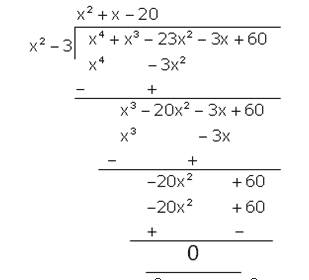Quotient q(x) = x2 + x – 20 = x2 + 5x – 4x – 20

= x(x + 5) -4(x + 5)

= (x + 5)(x – 4)

Other zeros of the given polynomial are the zeros of q(x)

Therefore, q(x) = 0 $\Rightarrow$ (x + 5)(x – 4) = 0

Or x = -5 or 4

Hence the zeros of given polynomial are $\sqrt{3}$, $-\sqrt{3}$, -5, 4

Question 13: Find all the zeros of (2x4 – 3x3 – 5x2 + 9x – 3), it Being given that two of its zeros are $\sqrt{3}$ and $-\sqrt{3}$.

Solution:

$\sqrt{3}$ and $-\sqrt{3}$ are the zeros of polynomial 2x4 – 3x3 – 5x2 + 9x – 3

Therefore, (x – $\sqrt{3}$)(x + $\sqrt{3}$) = x2 – 3 will divide the given polynomial completely

Dividing 2x4 – 3x3 – 5x2 + 9x – 3 by x2 – 3, we get: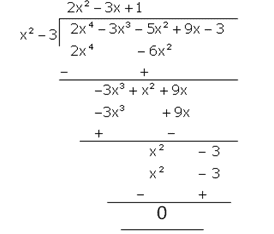Therefore, Quotient q(x) = 2x2 – 3x + 1 = 2x2 -2x –x + 1

= 2x(x – 1) – (x – 1) = (x – 1)(2x – 1)

Other zeros of given polynomial are given by

q(x) = 0 $\Rightarrow$ (x – 1)(2x – 1) = 0

Therefore, x = 1, ½

Hence, zeros of given polynomial are $\sqrt{3}$, $-\sqrt{3}$, 1, ½

Question 14: If two zeros of the polynomial (2x4 – 11x3 + 7x2 + 13x – 7) are (3 + $\sqrt{2}$) and (3 – $\sqrt{2}$), find other zeros.

Solution:

Sum of 3 + $\sqrt{2}$ and 3 – $\sqrt{2}$ = (3 + $\sqrt{2}$) + (3 – $\sqrt{2}$) = 6

Product of (3 + $\sqrt{2}$) and (3 – $\sqrt{2}$)

= (3 + $\sqrt{2}$)(3 – $\sqrt{2}$) = 9 – 2 = 7

Polynomial whose zeros are 3 + $\sqrt{2}$ and 3 – $\sqrt{2}$ is

x2 – (sum of zeros)x + (product of zeros) = x2 – 6x + 7

Dividing p(x) by x2 – 6x + 7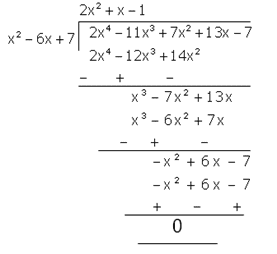Quotient = 2x2 + x – 1

Therefore, Other zeros of polynomial p(x) are also the zeros of q(x)

Therefore, q(x) = 2x2 + x – 1 = 2x2 + 2x – x – 1

= 2x(x + 1) – (x + 1) = (x + 1)(2x – 1)

q(x) = 0

$\Rightarrow$ (x + 1)(2x – 1) = 0

$\Rightarrow$ Either x + 1 = 0 or 2x – 1 = 0

$\Rightarrow$ Either x = -1 or x = ½

Therefore, The zeros of given polynomial p(x) are ½, -1, (3 + $\sqrt{2}$) and (3 – $\sqrt{2}$)

Question 15: Obtain all zeros of the polynomial (x4 + 4x3 – 2x2 – 20x – 15), if two of its zeros are $\sqrt{5}$ and $-\sqrt{5}$.

Solution:

$\sqrt{5}$ and $-\sqrt{5}$ are the zeros of the polynomial

x4 + 4x3 – 2x2 – 20x – 15

Therefore, (x – $\sqrt{5}$)(x + $\sqrt{5}$) = x2 – 5 will divide the given polynomial completely.

Dividing x4 + 4x3 – 2x2 – 20x – 15 by x2 – 5, we get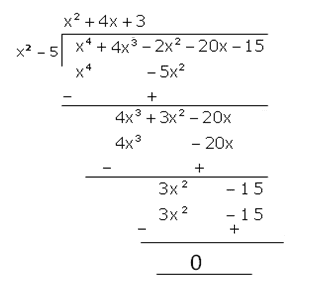Quotient = x2 + 4x + 3 = x2 + 3x + x + 3

= x(x + 3) + (x + 3) = (x + 3)(x + 1)

Other zeros of the given polynomial are the zeros of q(x)

Therefore, x = -3, -1

Thus, the zeros of the given polynomial are

$\sqrt{5}$, $-\sqrt{5}$, -3, -1

Q.16: Find all the zeros of the polynomial $(2x^{4} – 11x^{3} + 7x^{2}+13x – 7)$, it being given that two of its zeros area $(3 + \sqrt{2}) \; and \; (3 – \sqrt{2})$.

Sol:

The given polynomial is $f(x) = 2x^{4} – 11x^{3} + 7x^{2}+13x – 7$.

Since $(3 + \sqrt{2}) \; and \; (3 – \sqrt{2})$ are the zeros of f(x),

It follows that each one of ( $x + 3 + \sqrt{2}$ ) and ( $x + 3 – \sqrt{2}$ ) is a factor of f(x).

Consequently , $\left [ x – (3 + \sqrt{2} ) \right ] \left [ x – (3 – \sqrt{2} ) \right ] = \left [ (x- 3) – \sqrt{2} \right ] \left [ (x- 3) + \sqrt{2} \right ]$

= $\left [ (x – 3)^{2} – 2 ) \right ] = x^{2} – 6x + 7$, which is a factor of f(x)

On dividing f(x) by ($x^{2} – 6x + 7$), we get: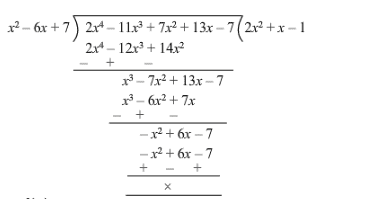$Therefore, f(x) = 0$

$\Rightarrow 2x^{4} – 11x^{3} + 7x^{2}+13x – 7 = 0 \\ \Rightarrow (x^{2} – 6x + 7) ( 2x^{2} + x – 1) = 0 \\ \Rightarrow (x + 3 + \sqrt{2}) (x+ 3 – \sqrt{2})(2x -1)( x + 1) =0$

$\Rightarrow x = -3 – \sqrt{2} \; or \; x = – 3 + \sqrt{2} \; or \; x = \frac{1}{2} \; or \; x = -1$

Hence, all the zeros are $\Rightarrow x = \left ( -3 – \sqrt{2} \right ) , \left ( -3 + \sqrt{2} \right ), \frac{1}{2} and -1$

Key Features of RS Aggarwal Class 10 Solutions Chapter 2 – Polynomials  Ex 2A (2.1)